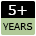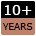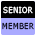Welcome to WebmasterWorld Guest from 3.85.214.0

Forum Moderators: open

Message Too Old, No Replies

Javascript form passing data between pages

I'm absolutely lost

Mauricio

11:14 am on Aug 31, 2003 (gmt 0)

New User from USjoined:Oct 16, 2014
posts:

I need to do something like this:

1.- The user must fill a form with three data: price (is fixen into a hidden input) quantity and a value from a dropdown menu; then a submit button.

2.- These data mut pass to a confirm page. The confirm page must show the price (quantityXprice) and
2.a) show a eight figures random number (a coupon number for the nex purchase)
2.b) an input for a coupon number (the script don't need to check this number; I do it manually)

I'm specially interested about passing the values. If you think some questions are too basical, please, post an URL or a piece of code.

See the code:

======================================
PAGE 1
======================================

<HTML>
<TITLE>Purchase form</TITLE>
<script>
<!--
function GoToConfirmPage(){
window.location.href = "confirm.htm";
//how to make javascript pass values to confirm.htm?;
}
//-->
</script>
<BODY>

<form name="purchase">
<input type="hidden" name="item1_price" value="14.90">
<input type="hidden" name="item1_desc" value="ACME item 1">
<p>ACME item 1</p>
<p>
<select name="item1_color">
<option value="">Color</option>
<option value="black">Black</option>
<option value="blue">Blue</option>
<option value="red">Red</option>
</select>
<input type="text" width="3" value="1">
</p>
<p><input type="submit" value="Purchase" onClick="GoToConfirmPage()"
</form>
</BODY>
</HTML>

======================================
PAGE 2
======================================

<HTML>
<TITLE>Purchase form</TITLE>
<script>
<!--
function Recalculate(){
if prev_coupon1 is filled then total_price_w/o_coupons = (total_price_w/o_coupons) -5
if prev_coupon2 is filled then total_price_w/o_coupons = (total_price_w/o_coupons) -10
if prev_coupon3 is filled then total_price_w/o_coupons = (total_price_w/o_coupons) -15
//obviously this is only a product of my lack of programing knowledge;
}
//-->
</script>
<script>
<!--
function OpenEmailClientAndSend(){
I'm still searching but I think I've seen this somewhere
//how to make javascript pass values to confirm.htm?;
}
//-->
</script>
<BODY>

<form name="confirm">
How to catch these values from the purchase page?</p>
<input type="text" name="item1_price" size="5" value="item1_price">
<input type="text" name="item1_desc" size="20" value="item1_desc">
<input type="text" name="item1_color" size="5" value="item1_color">
<input type="text" name="total_price_w/o_coupons" size="5" value="item1_price X quantity">
<input type="text" name="coupon" size="8" value="a random nunber">
</p>

<p>Do you have coupons from previous purchases? Type your numbers and then press "recalculate".<BR>
<input type="text" name="prev_coupon1"size="8" value="">
<input type="text" name="prev_coupon2"size="8" value="">
<input type="text" name="prev_coupon3"size="8" value="">
</p>

<p>The value of this box must be the total price w/o coupons and 5\$ less for each coupon number wrote.
It means<BR>
(total_price_w/o_coupons)<BR>
if prev_coupon1 is filled then -5<BR>
if prev_coupon2 is filled then -5<BR>
if prev_coupon3 is filled then -5<BR>
<input type="submit" value="Recalculate" onClick="Recalculate()">

<input type="text" name="total_price_final" size="8" value="Final price">
</p>

<p><input type="submit" value="Purchase" onClick="OpenClientEmailAndSend()"
</form>
</BODY>
</HTML>

storevalley

11:27 am on Aug 31, 2003 (gmt 0)

Preferred Memberjoined:Mar 4, 2003
posts:438

Hi Mauricio ...

Sounds like this would be far more easily done using CGI (make the form action a cgi script. This will pick up the details passed and generate a dynamic page containing them)

If you rephrase the question (how do I do this using CGI?) and post it here ...

[webmasterworld.com...]

... you'll probably get plenty of useful tips.

This assumes that your hosting company allows you to run CGI scripts, of course.

Mauricio

11:41 am on Aug 31, 2003 (gmt 0)

New User from USjoined:Oct 16, 2014
posts:

Thanks.

I'm gonna try with CGI.

My hosting company? Well... I have a dedicated server and am my own hosting company for I, me, mine. My customer service is very poor and the technical support is mantained by an ignorant. :)

storevalley

11:46 am on Aug 31, 2003 (gmt 0)

Preferred Memberjoined:Mar 4, 2003
posts:438

LOL ... Good luck Mauricio

claus

12:15 pm on Aug 31, 2003 (gmt 0)

Senior Memberjoined:June 15, 2003
posts:2412

Passing values between pages is as easy with JS as it is with serverside scripts. What you do is this:

1) On the sending page, construct this url using the form fields:

var url = 'www.example.com/receiving-page.htm?field1=x&field2=y&field3=z'

Then send it to the browser address line with something like this:

location.href = url;

2) On the receiving page, get the searchstring (the bit following the questionmark):

if (location.search){
var searchString = location.search;
}

Then you've got your fields and values passed on to the other page, now it's all in the string "searchString".

/claus

MonkeeSage

1:18 pm on Aug 31, 2003 (gmt 0)

Senior Memberjoined:July 26, 2003
posts:881

If you end up not being able to use a Perl CGI, it is possible w/ JS, but a little bit more of a pain...actually alot more.

You have to get the values from the forms on page 1, then when you request page two, append the values to the URL just like you would with a CGI GET, like page2.html?item=chicken&quant=3, then in page 2 you get the value of window.location.search.substring(1), which gives you everything after the "?", then you make an array and use the split method like split("&") and split("=")...

...so now you are thoroughly confused right? hehe, no worries he is a working example of what I mean.

page 1:

<HTML>
<TITLE>Purchase form</TITLE>
<script type="text/javascript">
<!--
function GoToConfirmPage(){
var price = document.getElementById("price").value;
var desc = document.getElementById("desc").value;
var color = document.purchase.item1_color.options[ document.purchase.item1_color.selectedIndex ].value;
var amount = document.getElementById("amount").value;
if (color) {
window.location.href = "testform2.htm?price=" + price +
"&desc=" + desc + "&color=" + color + "&amount=" + amount;
}
else {
}
}
//-->
</script>
<BODY>

<form name="purchase">
<input id="price" type="hidden" name="item1_price" value="14.90" />
<input id="desc" type="hidden" name="item1_desc" value="ACME item 1" />
<p>ACME item 1</p>
<p>
<select name="item1_color">
<option value="">Color</option>
<option value="black">Black</option>
<option value="blue">Blue</option>
<option value="red">Red</option>
</select>
<input id="amount" type="text" width="3" value="1" />
</p>
<p><input type="button" value="Purchase" onClick="GoToConfirmPage();" /></p>
</form>
</BODY>
</HTML>

page 2:

<HTML>
<TITLE>Purchase form</TITLE>
<script type="text/javascript">
<!--
// these are global
var price = "";
var desc = "";
var color = "";
var amount = "";

function populateFields() {
var hash = window.location.search.substring(1);
if (hash.length > 1) {
var itms = hash.split("&");
var vals = new Array();
var i;
for (i = 0; i < itms.length; i++) {
vals[i] = itms[i].split("=");
}
for (i = 0; i < vals.length; i++) {
if (vals[i] == "price") {
price = vals[i];
}
else if (vals[i] == "desc") {
desc = vals[i].replace(/\%20/g, " ");
}
else if (vals[i] == "color") {
color = vals[i];
}
else if (vals[i] == "amount") {
amount = vals[i];
}
}
if ((price) && (amount)) {
price = parseFloat(price * amount);
price = roundDp(price, 2);
var idx = price.toString().indexOf(".");
if ((idx!= -1) && (price.toString().substring(idx + 1).length == 1)) {
price = price + "0";
}
}
document.getElementById("price").value = price;
document.getElementById("desc").value = desc;
document.getElementById("color").value = color;
document.getElementById("amount").value = amount;
document.getElementById("fprice").value = price;
document.getElementById("rand").value = rand();
}
}
function Recalculate(){
var coup1 = document.getElementById("coup1").value;
var coup2 = document.getElementById("coup2").value;
var coup3 = document.getElementById("coup3").value;
var fprice = price;
if ((coup1) && (coup1.length >= 1)) {
fprice = parseFloat(fprice - 5);
}
if ((coup2) && (coup2.length >= 1)) {
fprice = parseFloat(fprice - 5);
}
if ((coup3) && (coup3.length >= 1)) {
fprice = parseFloat(fprice - 5);
}
fprice = roundDp(fprice, 2);
var idx = fprice.toString().indexOf(".");
if ((idx!= -1) && (fprice.toString().substring(idx + 1).length == 1)) {
fprice = fprice + "0";
}
document.getElementById("fprice").value = fprice;
// NOTE: ONLY substract 5 each time because the defecit is CUMULATIVE the way I have it set up
}
function OpenEmailClientAndSend() {
// no way to do this in JS that I know of...
}
// for rounding to nearest decimal point
function roundDp(num, dp) {
var r = Number("1e" + dp);
return Math.round(num * r) / r;
}
// for pseudo-random number
function rand() {
var now = new Date();
var num = parseInt(now.getSeconds() * 537873873).toString().substring(0, 9);
return num;
}
//-->
</script>

<form name="confirm">
How to catch these values from the purchase page?</p>
<input id="price" type="text" name="item1_price" size="5" value="" />
<input id="desc" type="text" name="item1_desc" size="20" value="" />
<input id="color" type="text" name="item1_color" size="5" value="" />
<input id="amount" type="text" name="item1_amount" size="5" value="" />
<input id="rand" type="text" name="coupon" size="8" value="a random nunber" />
</p>

<p>Do you have coupons from previous purchases? Type your numbers and then press "recalculate".<BR>
<input id="coup1" type="text" name="prev_coupon1"size="8" value="" />
<input id="coup2" type="text" name="prev_coupon2"size="8" value="" />
<input id="coup3" type="text" name="prev_coupon3"size="8" value="" />
</p>

<p>The value of this box must be the total price w/o coupons and 5\$ less for each coupon number wrote.
It means<BR>
(total_price_w/o_coupons)<BR>
if prev_coupon1 is filled then -5<BR>
if prev_coupon2 is filled then -5<BR>
if prev_coupon3 is filled then -5<BR>
<input type="button" value="Recalculate" onClick="Recalculate();" />

<input id="fprice" type="text" name="total_price_final" size="8" value="Final price" />
</p>

<p><input type="submit" value="Purchase" /></p>
</form>
</BODY>
</HTML>

But, since I don't know of any way to send mail using only JavaScript, this probably won't work for you and you will basically have to use a CGI script or PHP or something along those lines to finally send the page. But I figured I'd post this for posterity anyhow, in case someone else is ever wondering how to pass values between windows using only JS, or in case you want to use it up to the point where you actually send the page.

HTH
Jordan

--------
Edit:

Wow...that didn't seem like it took an hour! claus had me beat. :)